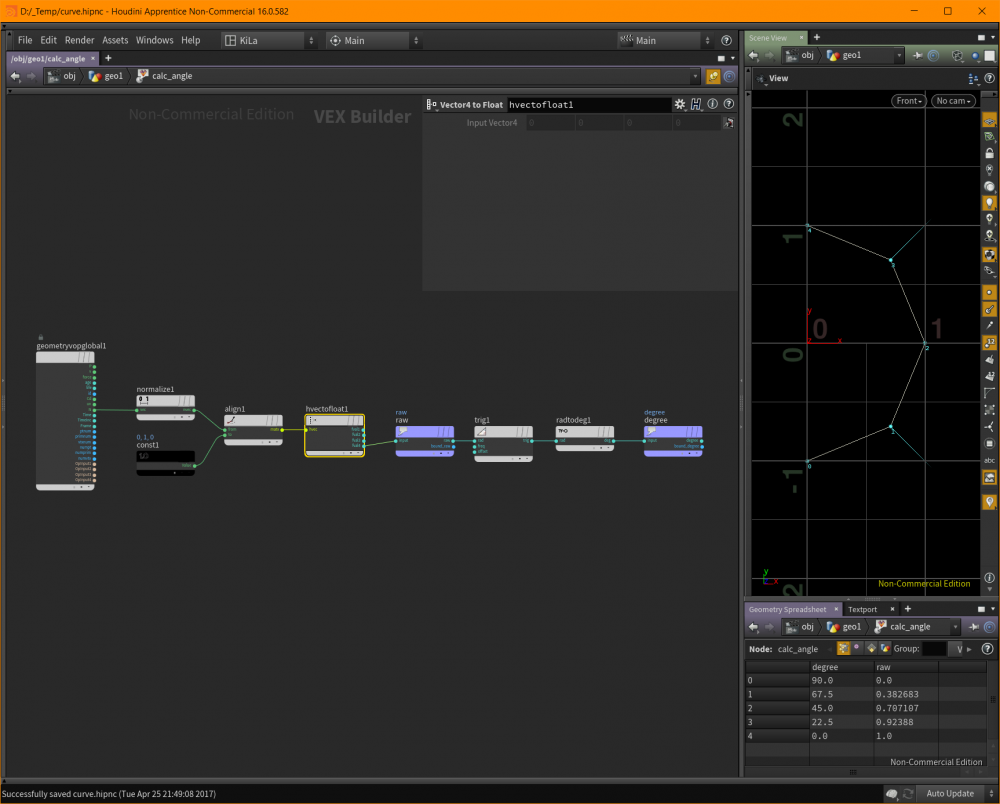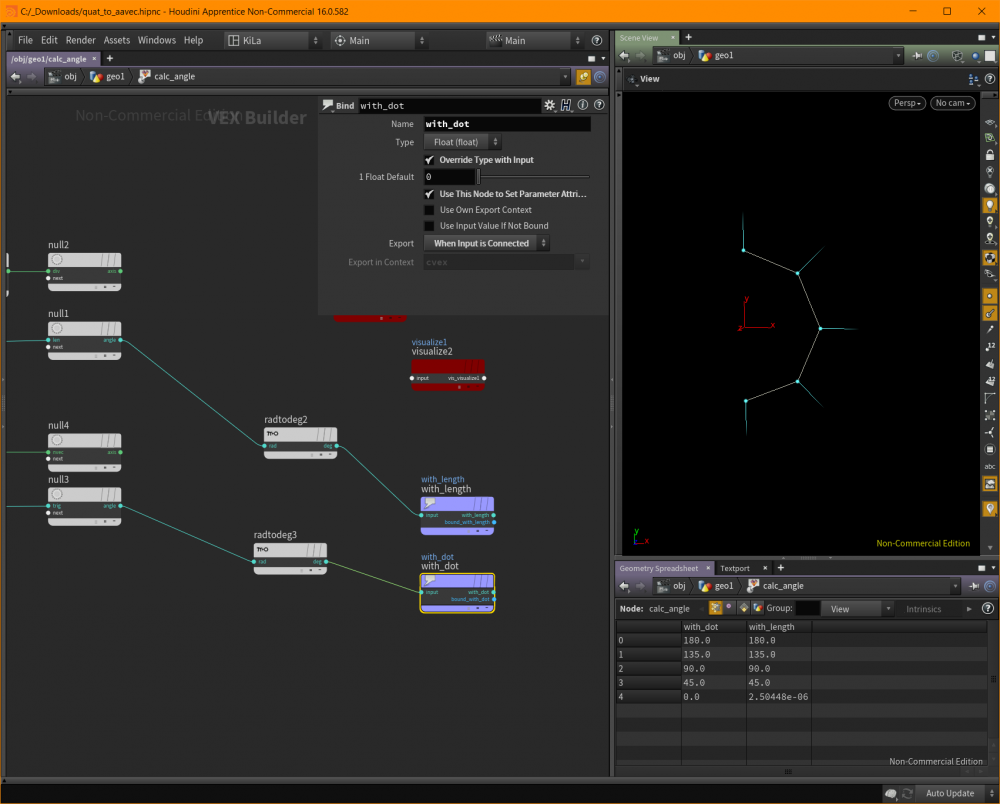# Using Align to calculate angle*axis between two vectors

## Recommended Posts

Hi, i am trying to calculate angle and axis between two vectors, using the Align VOP.. I has an option to export 'Angle Axis' - its help card says:

"This operator computes a matrix representing the rotation around the axes normal to two vectors (their cross product), by the angle which is between the two vectors. The resulting matrix maps the first vector onto the second. If both vectors have the same direction, the result will be an identity matrix. If the vectors are opposed, the rotation is undefined.

This can also output a quaternion representation of the transformation rather than matrix."

What does it mean?In the attached scene, i have an open circle which have some normals. Next i am trying to calculate the angle/axis between the normal an up vector, but the angles i get from the Align doesn't make any sense.

Cheer

-k

angle.hipnc##### Share on other sites

You still need to use Quaternion to Angle/Axis node after that. In result, you will get the axis multiplied by angle (in radians). Compute length to extract the angle. Normalize it (or divide it by length, same thing) to compute the axis. Alternatively, you can get axis by crossing vectors and normalizing the result and you can get angle by computing arccosine of dot product between vectors.

Edited by f1480187
##### Share on other sites

Haa, thank you Sir! Interesting tho - the length method seems to give 'wrong' angle for the first (0) point: 2.50448e-06. Whereas, the normal dot method gives the correct angle - 0.##### Share on other sites

That's equal to 0.00000250448 in normal representation. Pretty common, you shouldn't expect numbers to evaluate into ideal values in Houdini, and everywhere floating-point arithmetic is used. Search for "floating point imprecision", you'll quickly get the basic understanding of the problem.

## Join the conversation

You can post now and register later. If you have an account, sign in now to post with your account.
Note: Your post will require moderator approval before it will be visible.×   Pasted as rich text.   Paste as plain text instead

Only 75 emoji are allowed.

×   Your previous content has been restored.   Clear editor

×   You cannot paste images directly. Upload or insert images from URL.

×
×